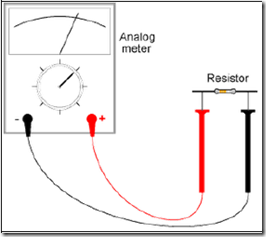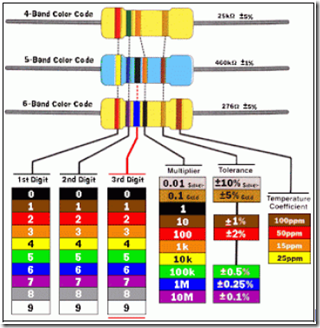" /> Ohm-meter Function | TN Elektro
Home > English > Ohm-meter Function

# Ohm-meter Function

Ohm-meter to measure the functioning of prisoners / resistance (resistance). In the techniques of electronics, custody / resistance (resistance) contains two meanings, first, the resistance (resistance) as a name for one of the electronic components namely resistance or resistor, and the second resistance given by conducting material (conductor) and / or materials semi conductor (semiconductor) contained in the electronic components of the electric current flowing in the same direction. Both have units expressed in ohms (Ω).

Based on the second point, we can say that: the electronic components that are made of conducting material (conductor) such as; resistors, capacitors, transformers, and windings (coil) and a half-conductive materials (semiconductors), such as; transistors, diodes, there are prisoners / resistance (resistance). Through the measurement of the value of resistance / resistance (resistance) contained in components that are out of sequence, we can find out whether a component can still function well and can still be used or damaged.

On Digital Multimeter, the measurement results can be read directly on the display screen, the Analog Multimeter, the measurement results prisoners / resistance (resistance) to read on board scale Ohm (Ω-kΩ). See figure 9.Figure 9. Measuring Prisoners (Resistance)
R = Resistivity / Resistance (Resistance)

To measure the value of resistance / resistance (resistance), switch the measuring range in the position Ω (Ohm). Limit measure (range) x1, x10, and xkΩ. Limit measure (range) for Ohm-meters from Multimeter vary, depending on the type and brand Multimeter. For example, type SP10D Sanwa Multimeter brands have measuring limit (range) x1, x10, and xkΩ. Multimeter brands Protek A803 has a measuring limit (range) x1, x10, x100, xkΩ, and x10 kΩ.

Measuring resistor
Resistor is a component that is widely used in the circuit input Kompo (vi). Limiting the ability of small value resistor unit Ohm (Ω) owned electronics. Its main function is to limit (restrict) the flow of electric current. Other functions as Resistor (R) voltage divider (voltage divider), which produces a forward bias voltage (forward bias) and a bias voltage backwards (reverse bias), as a potential generator output (vo), and potential.

Referring to Ohm’s law: I = V / R, the greater the value of resistance / resistance (R), the smaller the current (I) which can flow. The size of the unit value of which is owned by Ohm resistor can be calculated by looking at the tape (band) color contained in the resistor body. Following figure 10, if the first yellow ribbon, purple ribbon second, third band is brown, the fourth gold ribbon, the unit value of the resistor Ohm is 47 x 101 = 470 with a tolerance of 5%.

Please note, the yellow color indicates the number 4, the purple color indicates the number 7, the brown color indicates the numbers 1, thereby multiplying factor = 101, if the third band is red, a multiplying factor = 102, and so on. (See module on electronic components). For more details, refer to Figure 10.Figure 10. Value By Ohm Resistor Color Ribbons